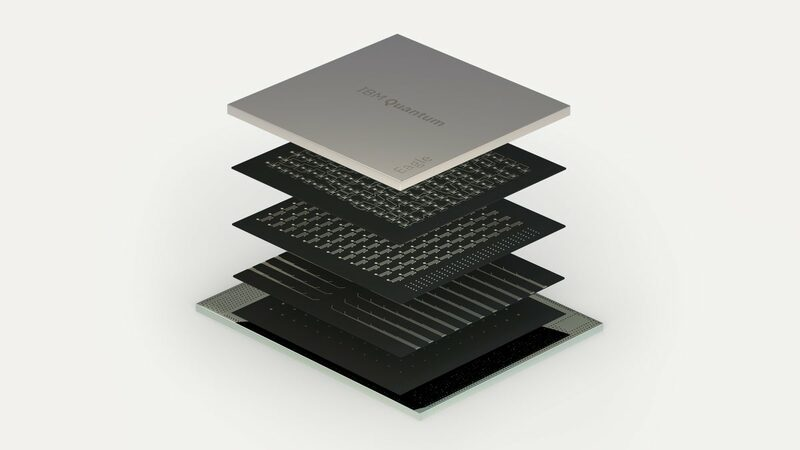Quantum Computers
Summary

Quantum computers are a new generation of computing devices based on the effects of quantum physics. Such devices using specialized quantum algorithms are capable of showing performance significantly superior to classical computers based on semiconductor technologies in some classes of tasks. In particular, an attacker, by implementing Shor's quantum algorithm on a promising quantum computer, will be able to effectively crack the most popular cryptographic systems with a public key at the moment.
More on the topic

Modern technologies, primarily computing, reach the limit of miniaturization, determined by the size of atoms. Classical models are not suitable for describing processes occurring at the atomic level, which led to a revolutionary development in understanding the laws of nature — the emergence of quantum physics. In turn, the development of quantum physics in the XX century made it possible to realize the concept of quantum computing, first expressed in the early 1980s (Yu. Manin, R. Feynman, P. Benioff).

Unlike a classical computer operating with elementary bits, each of which can take only two values: 0 or 1, a quantum computer works with finite sets of elementary states called qubits. A qubit has two basic states and can be in a state that is a linear combination of basic states with complex coefficients.

A simplified calculation scheme on a quantum computer looks like this: some initial state is recorded on the qubit system. Then, during the execution of the quantum program, the state of the system is changed by means of unitary transformations that perform certain logical operations. As a result, the state of the system is measured, which is the result of the work of the quantum program.

With the help of basic quantum operations, it is possible to simulate the operation of ordinary logic elements from which classical computers are built, thereby, a quantum computer in future is able to solve any problem solved on a classical computer, including cryptanalysis problems.

In turn, the operation of a quantum computer can be emulated using a classical computing system, for example, using graphical coprocessors, but such emulation is possible only for systems with a small number of qubits due to the exponential growth in the number of necessary logic elements

In some cases, the use of a quantum algorithm can give a significant increase in the efficiency of calculations. The most critical application of quantum computing for information security tasks is Shor's algorithm for factorization and discrete logarithm, which gives an attacker the ability to effectively crack most of the public-key cryptosystems currently in use.
Building a «full-fledged» quantum computer capable of solving applied problems is a fundamental scientific and engineering task. At the moment, there are already working research prototypes of quantum computers with about 100 connected qubits (for example, the IBM Eagle quantum processor, which appeared at the end of 2021, has 127 qubits). For a number of difficult computational problems «quantum superiority» has already been demonstrated ― an effective solution to a problem previously considered inaccessible to classical computers.Quantum processor IBM Eagle Ⓒ IBM, 2021
Modern quantum computers usually use one of the following basic technologies:
• solid-state quantum dots on semiconductors;
• superconducting elements;
• ions in Paul vacuum traps (or atoms in optical traps);
• mixed technologies;
• optical technologies.
Narrowly focused quantum calculators stand apart, such as quantum simulators designed to solve specific problems from the field of modern chemistry, as well as multi-qubit solutions from D-Wave, which effectively solve only some optimization problems, but are unsuitable for practical cryptanalysis.

The main challenges for creating quantum computers are::
- instability of quantum systems;
- sensitivity to the environment;
- accumulation of errors in calculations;
- difficulties with initial initialization of qubit states;
- difficulties with the creation of multi-qubit systems.

However, taking into account the general trends in the development of state-of-the-art technology, as well as the growing interest of business, industry and states in the field of quantum computing (and, accordingly, the growing volume of investments in this area), QApp experts assume with high probability the appearance of a quantum computer capable of effectively solving the problems of applied cryptanalysis in the horizon of 2028-2030.

How can we effectively counteract the quantum threat today?

In order to effectively counteract the quantum threat in your business, you should use solutions based on post-quantum cryptography. QApp has expertise and its own developments capable of protecting valuable data from hacking using a quantum computer.

In order to minimize the business risks associated with the development of quantum computing, you can: